﻿ Analysis of Determinisitic Aritificial Intelligence for Inertia Modifications and Orbital DisturbanceInternational Journal of Control Science and Engineering

p-ISSN: 2168-4952    e-ISSN: 2168-4960

2018;  8(3): 53-62

doi:10.5923/j.control.20180803.01### Analysis of Determinisitic Aritificial Intelligence for Inertia Modifications and Orbital Disturbance

Keith Lobo1, Jonathan Lang1, Anthony Starks1, Timothy Sands2

1Department of Mechanical and Aerospace Engineering, Naval Postgraduate School, Monterey, USA

2Department of Mechanical Engineering, Stanford University, Stanford, USA

Correspondence to: Timothy Sands, Department of Mechanical Engineering, Stanford University, Stanford, USA.
 Email: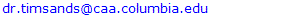Abstract

Recent advances in the pioneering field of deterministic artificial intelligence applied to space systems energize this manuscripts evaluation of two novel aspects: sudden changes to moments of inertia (e.g. ejection or unplanned retention of an apogee kick motor), and disturbance rejection efficacy in the face of gravity gradient and atmospheric torques generated by density gradients. Encasing this research is critical evaluation of minimal error in attitude control in the face of these aspects. The errors are found to favourably compare to the capabilities demonstrated in the recent literature by deterministic artificial intelligence, and this key contribution allows researchers to conclude from this manuscript the strong efficacy in the face of retention of apogee kick motors, even in the presence of realistic orbital disturbances. Numerical modeling supports simulation experiments that also include requisite disciplines of space phoronomics and autonomous trajectory generation (proposed by companion manuscripts currently under peer review) yielding iterative analysis to establish efficacy. Some of the main findings include validation of the application of deterministic artificial intelligence techniques to attitude control in space in addition to elaboration of the coincident, fleeting effects of gravitation disturbances and atmospheric torques as a function of orbital altitude.

Keywords: Artificial intelligence, Non-stochastic, Deterministic, Control systems, Autononomy, Intelligent systems, Space robotics, Feedforward, Disturbance modeling, Automatic trajectory generation, Phoronomics

Cite this paper: Keith Lobo, Jonathan Lang, Anthony Starks, Timothy Sands, Analysis of Determinisitic Aritificial Intelligence for Inertia Modifications and Orbital Disturbance, International Journal of Control Science and Engineering, Vol. 8 No. 3, 2018, pp. 53-62. doi: 10.5923/j.control.20180803.01.

### 1. Introduction

Contemplating autonomously overcoming dynamic disturbances in space and unexpected changes in mass inertia may be addressed by the general methodology of deterministic artificial intelligence which stems from a lengthy heritage that harkens back at least 1830 with Chasle’s theorems of motion Phoronomics  followed shortly afterwards in 1846 with Newton’s Principia , continuing in 1775 with Euler’s formulations  with relatively immediate expansion throughout the nineteenth [4-7], justifying the later adopted of Tait angles to describe kinematics; and then followed in twentieth centuries [8-30] with a particular renaissance in the late twentieth century accompanying the race between the then-Soviet Union and the United States for spaceflight and its accompanying application toward nuclear deterrence, where considerable lessons from that period (both technical and non-technical) have been recently expressed in subsequent literature [31-69]. From this distinguished lineage, a contextual aspect has resurfaced with the rise of the looming threat of great power conflict in a context of many world powers also becoming space-faring nations. Accompanying this political context once again, the modern age sees impetus for significant technological advancement, this time towards autonomous, fault-tolerant, damage-tolerant control systems that include online state estimation, adaptive and non-adaptive feedforward controllers for trajectory tracking and disturbance rejection; and autonomous trajectory generation together forming intelligent space systems that no longer require phalanx’s of ground personnel to operate space systems. This article discusses recent research in the underlying technologies of rotational mechanics, modern kinematics (in an information-age context), and disturbance modeling for autonomous robust rejection, with particular emphasis on the italicized topics. From the recent literature, we see that [46-55] the deterministic artificial intelligence methodology can counter mass inertia disturbances up to 90% while maintaining single-digit to decimal degree accuracy  where this key metric will be used in a novel comparison of performance in a realistic disturbance environment amidst retention of significant mass vis-à-vis an apogee kick motor. Nonlinear stability is demonstrated via lyapunov-energy function in  and using phase portraits in .

### 2. Materials and Methods

#### 2.1. Rotational Mechanics Fed by Autonomous Trajectory Generators

Initial modelling developed a commanded spacecraft body movement, and subsequently translates the commanded-movement into input torque commands applied to the spacecraft’s body. Kinematics subsystems expressed the quaternion and direction cosine matrix (direction cosine matrix) and used to find Euler Angles for attitude with respect to an inertial reference frame. The simulation topology of is shown in Figure 1.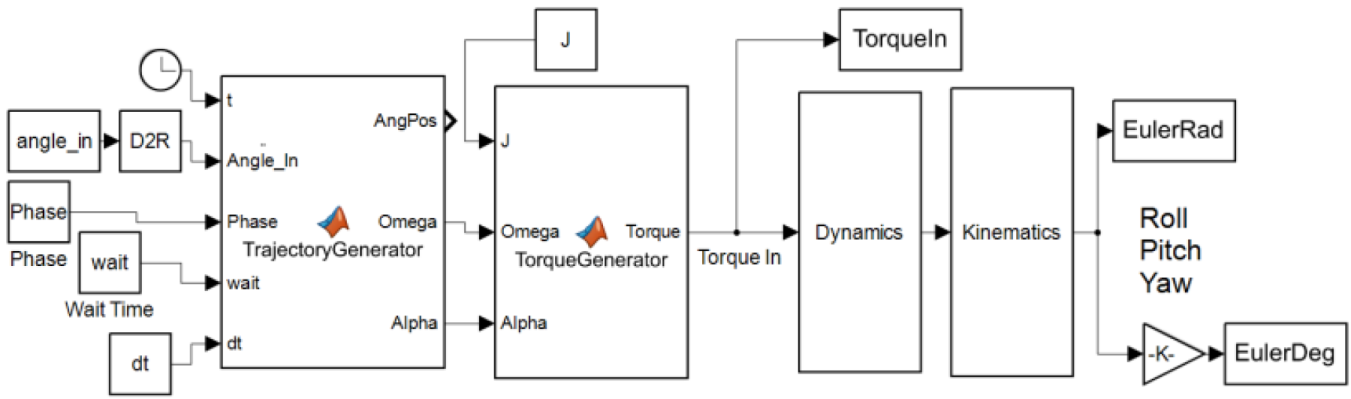Figure 1. Simulink Model Topology
The effects of varying time-steps and commanded maneuver-time in feedforward control (everything to the left of dynamics block in figure 1) are investigates, in particular emphasizing the numerical accuracy and impacts on computational time for various methods.

#### 2.2. Dynamics

Dynamics is synonymous with Mechanics. Newton called dynamics the science of machines which may be divided into two parts: statics (later called kinematics) and kinetics . Chasle’s Theorem  articulates how a complete description of motion may be described screw displacement comprised of translation in accordance with Newton’s Law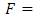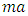and rotations in accordance with Euler’s Moment Equations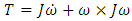(both equations named subsequent to Chasle’s expression), where m is the mass of the object and [J] is a matrix of mass moments of inertia very well explained by Kane . Thus, unplanned changes in the components of [J] are deleterious since the control force F applied as a torque is designed for the formerly assumed [J].
Investigation of motion without consideration of the nature of the body moved or how the motion is produced is called Phoronomics, or “the laws of going”, or more commonly but less properly kinematics , to be briefly discussed in section 2.3.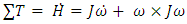(1)
The Dynamics block of the system topology in Figure 1 contains equation (1) elaborated in equations (2) and (3), while the calculated command torque is provided by equation (4) in the Feed Forward Control section fed by equations (25)-(28) in the Trajectory Generator (the far left inputs of the topology) to be elaborated in section 2.4.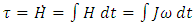(2)
In the spacecraft’s dynamics captured in equation (1) and (2),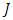represents the moment of inertia,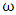is the angular velocity, and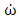is the angular acceleration. In (2), the angular momentum, defined as H, with its change defined as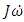. The additional component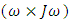in equation (1) represents the coupled motion of the spacecraft when there is rotation about more than one axis. This coupling creates induced motion about the other axes and is proportional to the mass distribution in the inertia matrix.
To derive the angular velocity of the body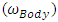fed from Dynamics to Kinematics, we multiply moment of inertia (J) by the time derivative of angular velocity, i.e., angular acceleration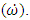This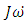term is multiplied by the inverse of J and then integrated as per equations 2 and 3. Afterwards,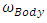is used to obtain the spacecraft attitude’s Euler Angles.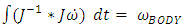(3)
Any change in Moment of Inertia will impact the ability of a spacecraft to execute commanded maneuvers. This paper studies the impact of a discrete change in moment of inertia on the ability of a numerically modeled (rigid body) spacecraft to successfully implement a commanded Euler angle maneuver. A sudden change in moment of inertia simulates large discrete changes, resulting from scenarios in which there are major changes to the spacecraft’s mass or mass distribution, such as orbital debris damage, jettison of a large component or stage, or catastrophic failure of major mass components. The model was used to simulate a single discrete change in the moment of inertia while implementing a 30° yaw maneuver.
Equation (4) contains the idealized feedforward control that forms the basis of deterministic artificial intelligence . It is called idealized, because the control is formulated from equation 1 with moments of inertia assumed from the last known values, while the motion-states are provided by a desired tractor (thus the subscript d) in equation (4). This dependency also highlights the importance of the desired trajectory to be discussed in section 2.4.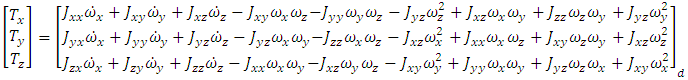(4)
Equation 4 is the foundational basis of deterministic artificial intelligence.
##### 2.2.1. Change in Moment of Inertia
Change in the moment of inertia in the spacecraft is a common occurrence. Example scenarios include, the shifting of an internal component of a spacecraft during launch, the burning of fuel in an onboard tank, or even damage of the spacecraft due to debris. These changes in mass or mass distribution cause a change in the moment of inertia. The moment of inertia studied here results from (for example) a catastrophic failure of an apogee kick motor (apogee kick motor). The model assumes an idealized, diagonalized moment of inertia matrix at the start of the maneuver: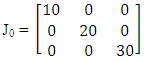(5)
The moment of inertia after the simulated apogee kick motor failure is: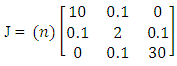(6)
The latter moment of inertia assumes failure with substantial distributions of the effects in the xy and yz planes. Various iterations of n from 1 to 7 were run to test scaling of J. These methodologies require examination due to the inherent errors in an actual spacecraft’s measurement apparatus. No onboard system can ever measure a spacecraft’s angular position, velocity, or acceleration at infinite precision. Additionally, any disturbances accounted for in the coarse control from our feed forward design will still require additional feedback control for robust, fine tuning. As such, it may be more prudent to embrace a small amount of course control error in order to speed up overall computation time. In order to assess these methodologies, a SIMULINK model for each Trajectory Generator method was created and their outputs were compared for error and computation time.

#### 2.3. Kinematics

Consensus terminology has converged to refer to sequential rotation sequences (e.g. xyz or 123) as aerospace sequences about non-repeating axes (also referred to as “Tait-Bryan angles”), while the orbit sequences have an axis repeated in the rotation sequence (e.g. xyx or 121, also referred to as “proper Euler angles”), ; with one non-repeating sequence in particular (commonly called either a 321 or 123 sequence) has become the ubiquitous aerospace sequence. With the rise of digital computation in the Information Age,  critically evaluates the options seeking diverging truths for the modern times addressing such questions as: Is the ubiquitous aerospace sequence (123 or alternatively 321) the best rotation sequence for the information age dominated by the digital computer? Evaluation was be driven by two figures of merit in : 1) mean and standard deviations of errors indicating how well each rotation sequence represents true roll, pitch, and yaw-angles, and 2) computation time to reveals relative numerical superiority in the context of digital computers of the current state of the art. Analysis and results demonstrate the fact that 321 and 123 rotation sequences result in disparate errors and computation time, with the former being relatively superior. Furthermore, the 123 rotation was significantly slower than all the other rotations. The symmetric rotations were on average slower than the non-symmetric rotations, despite the same mathematical process and number of steps to solve for the Euler Angles. Lastly, the fastest non-symmetric rotation was the 321 and the fastest symmetric was the 232, slightly faster than the 121 rotation. Taking all direction cosine matrix rotations into account, the 232 rotation was the fastest.
##### 2.3.1. Direction Cosine Matrices
The first step of the model creation was the implementation of a direction cosine matrix (direction cosine matrix) to numerically represent rotations about a set of axes to project a starting frame onto a desired reference frame. Figures 2 and 3 provide visual depiction of the process driven by equations (7) through (10). Each direction cosine matrix equation takes an axial rotation as depicted by the series of rotations in Figure 2 and represents it as an orthonormal matrix consisting of sines, cosines, zeroes, and ones per the trigonometry rules shown in Figure 3.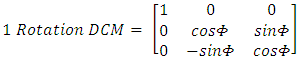(7)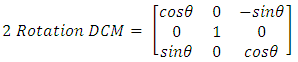(8)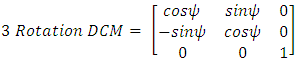(9)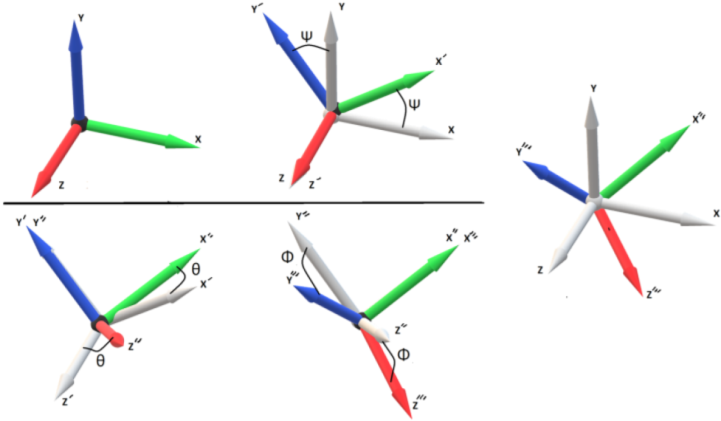Figure 2. Simple 3-2-1 Rotation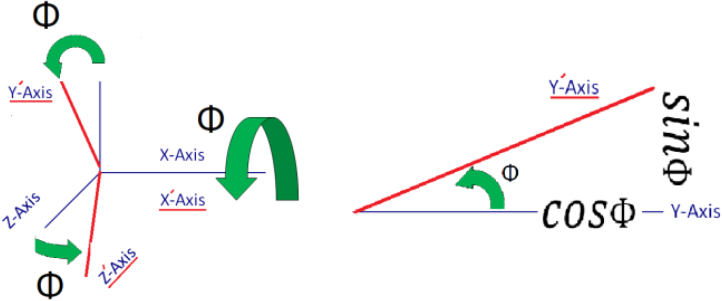Figure 3. Depiction of a Φ Rotation about the X axis
For more complicated movements, direction cosine matrixs are multiplied together resulting in a more intricate matrix. Equation (12) is an example of this for a 3-2-1 Rotation. This direction cosine matrix sequence and others are provided a more in depth treatment in Ref .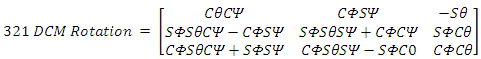(10)
*Note that the ‘C’ and ‘S’ characters are shorthand for sine and cosine respectively.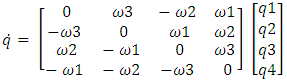(11)
The other major workhorse of the model’s kinematics is the orthonormal quaternion matrix, which accomplishes the same feat as the direction cosine matrix but with intrinsic divide by zero protection. The particular quaternion calculation format implemented in the model can be seen in equation 2-5. To find the quaternion solution for time from equation 2-5, it is a simple matter of initializing the quaternion vectors with an orthonormal set (i.e, [0,0,0,1]T) and integrating the output. The final matrix utilized, shown in equation (12), is an equation equivalent to the 3-2-1 Rotational matrix equation of (10) but written in terms of quaternion elements. This quaternion centric 3-2-1 Rotation is depicted below in equation (12).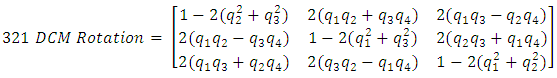(12)
Implementation in SIMULINK models equation (12) but for calculation purposes takes advantage of (10) to discern the Euler angle outputs. Equating terms in equations (10) and (12) leaves yields solutions for Euler angles in equations (13) through (15).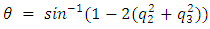(13)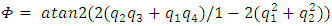(14)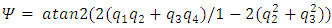(15)
##### 2.3.2. Quaternions
The angular velocity of the spacecraft, in the inertial frame, can be found by dividing the angular momentum produced in equation (3) by the moment of inertia.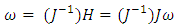(16)
To represent the motion of a rigid body (such as a spacecraft) in inertial space, the angular velocities can be expressed as quaternions (denoted by the letter q), using a first order differential equation (17). These are quantities which provide the orientation of a single axis (the eigenaxis) which remains unchanged in both the inertial reference frame and the body reference frame. The quaternion vector is a 4 by 1 vector: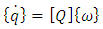(17)
When angular velocity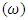is known, the time invariant solution of the ordinary differential equation in (17), produces a [4x1] dimension quaternion vector (q):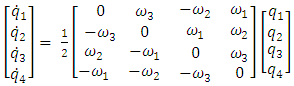(18)
##### 2.3.3. Direction Cosine Matrix-Quaternion Equivalencies
A combined three axis rotation of a rigid body can be expressed with quaternions. The first three elements detail the translation of the three axes, such that the coordinates orient the Euler axis in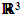space. The fourth quaternion details the rotation of the Euler axis about itself. A 3-2-1 rotation can be constructed with quaternions using (19):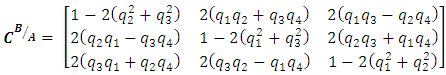(19)
##### 2.3.4. Generation of Euler Angles from Direction Cosine Matrix
The direction cosine matrix can also be found by the constructing a rotational matrix from the three angles of rotation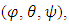which normally represent rotational movements of pitch, roll and yaw using component rotations (e.g. equations (7), (8), and (9)).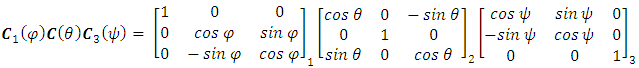(20)
The combined sequence of rotations (CB/A) can be constructed as a single matrix as expressed in equation (10) with the understanding the matrix represents a rotation from basis reference frame A to frame B (e.g. from body to inertial frames) per equation (21).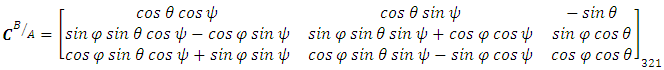(21)
By comparing (19) to (19), it is possible to determine the Euler angles for the 3-2-1 rotation sequence per equations (22) through (24) which are equivalent to equations (13) through (15).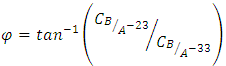(22)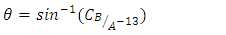(23)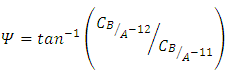(24)

#### 2.4. Trajectory Generation

The aforementioned feedforward architectures rely on modeling of dynamic system equations, and furthermore the control equations will be seen to necessitate formulation of a desired full-state trajectory: at least angular velocity and angular acceleration trajectories to formulate the control in addition to angular position to formulate the error.
To accurately model spacecraft maneuvers a standard sine curve (equation (25)) is used to generate a desired trajectory to populate the Trajectory Generator in figure 1.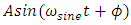(25)
where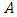is the amplitude of the maneuver or the desired angular displacement of the spacecraft. The rate at which the spacecraft completes the maneuver is the frequency of the spacecraft denoted as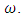The phase on the sine curve, used to shift the trajectory curve for smooth onset, is denoted by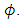Using the Commanded Euler Angle and the Desired Slew time in conjunction with equation (25), the commanded maneuver is translated into a trajectory using various sine curves. Equation 25 above is expanded into equations (26) through (28) below for investigations of moment of inertia changes in the dynamics, while equation 26 below is used to investigate and compare numerical methods.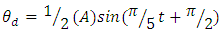(26)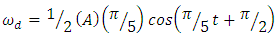(27)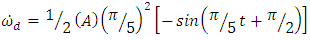(28)
Equations 26-28 feed equation 4, the foundational basis of deterministic artificial intelligence. Using equation (26), the first and second time derivatives provide the commanded angular velocity and acceleration in equations (27) and (28). To ensure system stability, a quiescent time period is provided before the maneuver. The commanded trajectory is implemented over five seconds, after five seconds of quiescent time used to verify correct model operations. The simulation is continued for five seconds after the commanded maneuver to provide sufficient settling time. The Trajectory Generator takes in a commanded body angle and then uses a sine wave in order to approximate the commanded maneuver.
The simulation model’s Trajectory Generator in Figure (1) contains equations 29 through 31 to approximate the commanded maneuver for Angular Position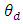, Angular Velocity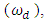and Angular Acceleration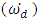via the MATLAB sine wave function.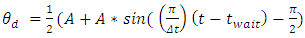(29)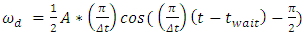(30)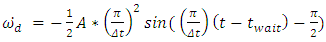(31)
Equation 2-12 models the input command, where A is the maneuver’s commanded angle. The base frequency of the sinusoid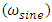is (π/Δt) where (Δt) is the desired maneuver time. The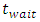term allows for a quiescent period and -π/2 term allows for a proper phase shift to implement the sinusoidal half period. Equations (30) and (31) are just successive derivatives of equation (29) used to generate angular velocity and acceleration which are fed into the ideal feedforward control equation  which resides in the torque generator block of Figure 1. This produces an output torque which drives the dynamics.

#### 2.5. Disturbances

Aerodynamic forces applied over a spatial gradient (stronger at lower altitudes in relatively thicker air) produce an unplanned disturbance torque inducing unplanned rotations. Similarly, Gravity forces are stronger in orbits closer to the center of the earth, resulting in a force gradient generating another disturbance torque producing unplanned rotations. Adaptive and non-adaptive feedforward architectures relying on system identification and disturbance identification [46-59] effectively counter the disturbances and reduce or eliminated the resulting rotational motion. Modeling and analysis are performed in this manuscript for comparison to the efficacy of these methods revealed in the literature.
The ideal feedforward controller must account for the disturbances  effecting the spacecraft. Given the relative strength of the gravity gradient and aerodynamic forces in Low Earth Orbit (LEO), the torques from these forces was evaluated for comparison to the ability of fault-tolerant control methods in literature [46-59] to accommodate and reject such.
##### 2.5.1. Aerodynamic Torque
This torque is a result of aerodynamic drag forces acting disparately on the moment arm between the center of gravity and center of pressure on the spacecraft axes of the spacecraft’s body frame (expressed in the Direction Cosine Matrices (direction cosine matrix)) :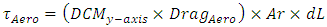(37)
where in equation (37), Ar represents the surface area of the spacecraft in each axis and dL represents the elemental distance from the center of pressure along that axis.
Highly prevalent in low-earth orbits, gravitational torque (resulting from a gravitational force gradient) detailed in equation (38), acts strongly about the y-axis and z- axis (extractable from the direction cosine matrix).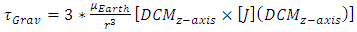(38)
Gravity gradient torque creates a restoring couple when the spacecraft is displaced from equilibrium, driving the spacecraft back towards its originally stable position. The disturbance torque is accommodated by adjusting the feed forward torque controller to compensate for them: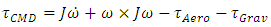(39)

### 3. Results

This section describes the results of each of the major areas of investigation. Dynamics are elaborated with an analysis of the efficacy and impacts of faults resulting in unplanned retention of a spacecraft’s apogee kick motor. Evaluation of computational efficiency  produced guidance to researchers about minimum step-size, which was validated in section 3.2.1 of this manuscript. Meanwhile, disturbance environments are analyzed for comparison to the autonomous-rejection capability elaborated in the literature [46-59].

#### 3.1. Dynamics and Changes in Moments of Inertia

To model the effects of a discrete change in moments of inertia, owing to a catastrophic failure as described in section 2.2.1, a Simulink model was used. The Simulink model (topology in Figure 1) consists of five major blocks: Trajectory, Dynamics, Kinematics, Disturbances and direction cosine matrix to Euler Angles. The Trajectory block implements equations (29), (30), and (31) to convert a commanded Euler angle into a desired torque to be fed forward into the Dynamics block. The Dynamics block implements equation (4), converting the inputted torque into an angular velocity in the body frame. The Kinematics lock implements equations (10) - (15), converting angular velocity into quaternions and a direction cosine matrix. The direction cosine matrix to Euler Angles block implements equations (18), (19) and (21) – (24) to convert the direction cosine matrix into Euler angles in the inertial frame. Finally, the disturbances block implements (37) - (38) to determine disturbance torques due to gravity gradient and aerodynamics forces. The Simulink model uses a step size of 0.01s and the ODE4 Runge-Kutta Solver as determined by  and validated here in section 3.2.1. For the moment of inertia matrix in (5), a 30° yaw produced the quaternion displacement shown in Figure 4: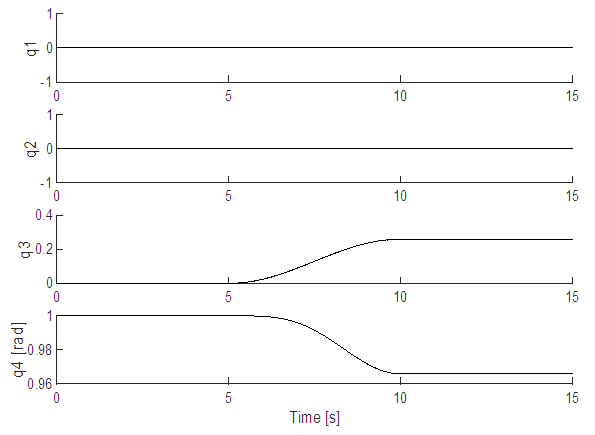Figure 4. Quaternions for the initial nominal [J]
With the loss of the apogee kick motor and the adjustment to a new moment of inertia (6) the quaternions result in Figure 5.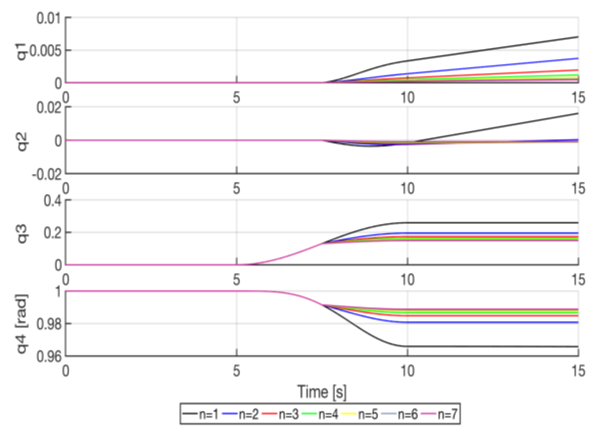Figure 5. Quaternions for the adjusted [J] post apogee kick motor failure (for various multiples of [J] in equation (6))
Figures 4 and 5 illustrate the impact of the change in moment of inertia, as coupling induces rotation about the other two axes, commencing at the 7.5s mark, after apogee kick motor failure mid-maneuver.
Figure 5 also shows the convergence of the quaternions to erroneous steady-state values as the moment of inertia post-failure is larger than planned in the control. The first two quaternions (proportional to roll and pitch) correctly converge towards zero for the commanded yaw-only maneuver while the third quaternion (proportional to yaw) converges on 0.15 and the fourth quaternion (proportional to the rotation angle) converges on 0.99; illustrating a dampening effect of the moment of inertia via nonlinear coupling as determined by the ratios of mass distributions within the inertia matrix in equation (6).

#### 3.2. Trajectory Generation Computational Investigation

##### 3.2.1. Solver/Integrator Step-size
The simulation was run with a fixed step solver using the Runge-Kutta method. In order to determine an appropriate step size for the solver three separate cases were run with three different step sizes. Using 1(blue), 0.1(red) and 0.01(yellow) as examples it became apparent that 0.01 was roughly ideal, as Figure 6 displays correct trajectories for the expected 30 degrees in the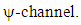Finer iteration followed the previous investigation of computational efficiency.
For the purpose of model verification, commands are initially held constant at zero resulting in zero torque to allow us to evaluate model operation during a quiescent period. With zero input torque, equation (1) shows us that the change in angular momentum should be zero as well; therefore the motion states should not change. After a five second quiescent period, a 30° yaw maneuver was commanded of each model and the maneuver was conducted over a ten second period. Afterwards the output Euler Angles should remain constant with the spacecraft’s new attitude. The results of this test were plotted in Figure 6. Additionally, note that the model was setup with a diagonalized J matrix per equation (5). The roll and pitch Euler Angles remained at zero, as they should have, and as such are not shown.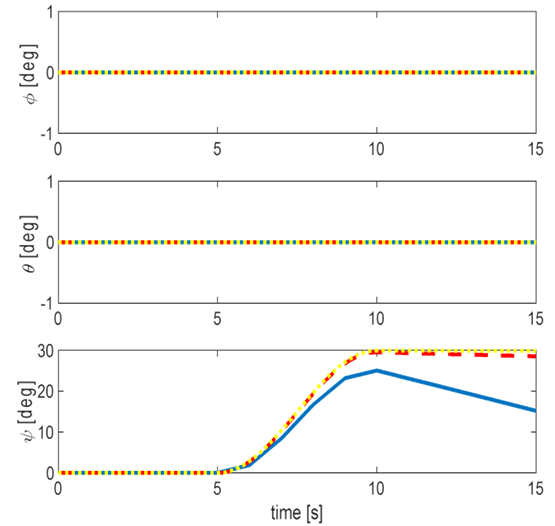Figure 6. The Euler angles after a 30 degree yaw, computed using the three step sizes

#### 3.3. Investigation of Disturbances

The simulation began with an initial angular velocity of [0 0 0]. Using (3), the angular velocity was input into the kinematics for a maneuver of 30 degrees in the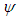direction. The maneuver is set up to begin 5 seconds into the simulation and take a total of 5 seconds to complete. Figure (7) shows the four separate altitudes corresponding to Table 1 that the aero torque is tested at and the respective atmospheric densities at those heights. Figures 8-11 shows the differing effect of the aero torque on the movement of the spacecraft corresponding to table 2. The maneuver looks increasingly different as the distance from Earth increases as numerically assessed in Table 2. Table 2 shows the numerical decrease in the effect of the aero torque over an increasing distance. In the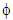channel, the error decreases from 0.0034 to 5.58e-10 over a distance of 364km.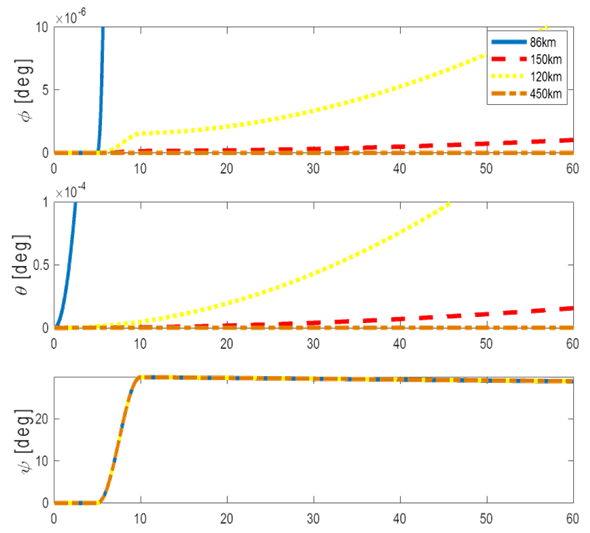Figure 7. The Euler angles for a 30 degree yaw maneuver. Data represents angles at each of the four different altitudes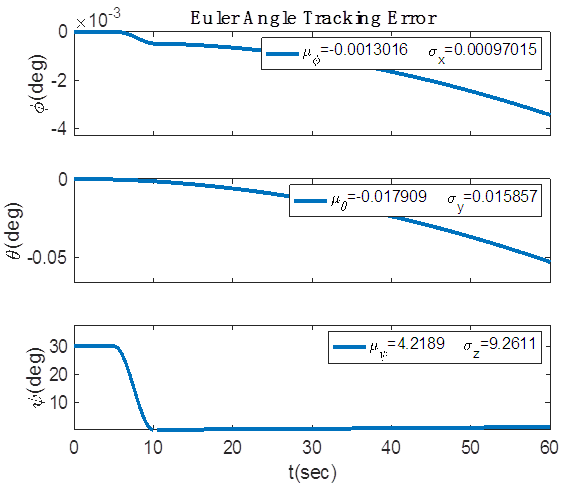Figure 8. Error between input trajectory and Euler angles. 86km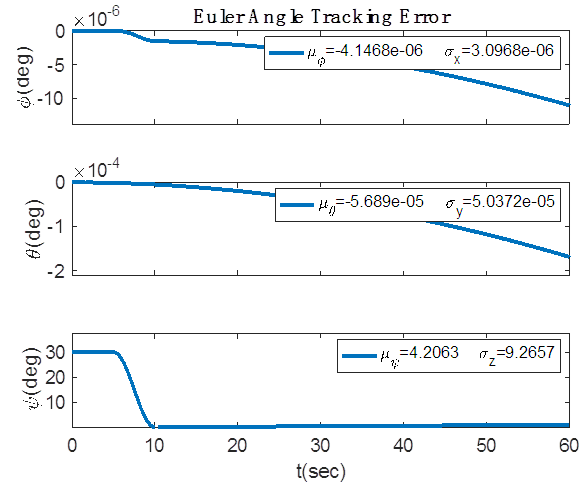Figure 9. Error between input trajectory and Euler angles. 120km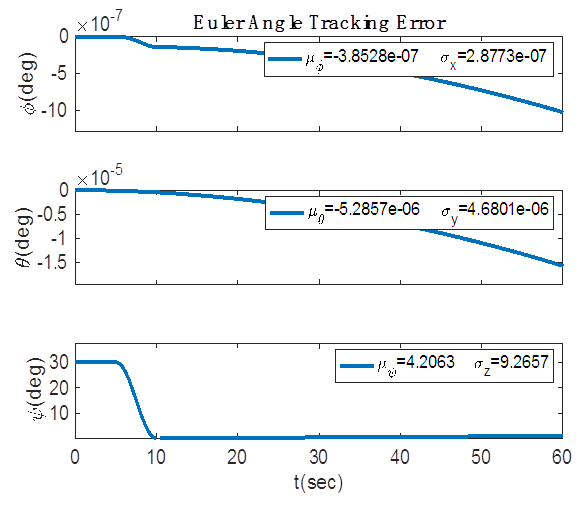Figure 10. Error between input trajectory and Euler angles. 150km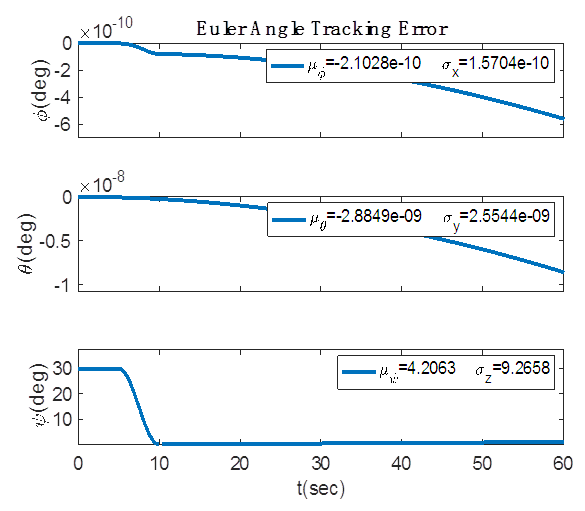Figure 11. Error between input trajectory and Euler angles. 450km
 Table 1. Atmospheric densities at four specific altitudes used for calculation of the Aero Torque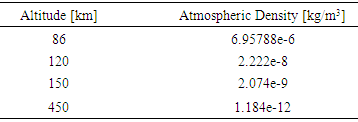Table 2. Euler angles error at the end of the maneuver for each altitude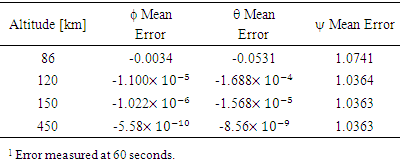### 4. Conclusions

Investigations of unplanned changes in the moment of inertia of space systems revealed resulting motions that were within tolerance of current damage-tolerant algorithms [46-55], [66-67] that rely heavily on these types of modeling efforts to devise intelligent feed-forward control algorithms to anticipate and negate the deleterious effects. The errors are found to favourably compare to the capabilities demonstrated in the recent literature by deterministic artificial intelligence, and this key contribution allows researchers to conclude from this manuscript the strong efficacy in the face of retention of apogee kick motors, even in the presence of realistic orbital disturbances.
Analysis of disturbances confirmed the decreasing effects of the atmosphere with increasing altitudes are predictable, and the assumption that it would decrease significantly was proven. In fact, the decrease was even greater than expected at an even faster rate than expected. It should be noted that the 30 degree maneuver was still completed in the amount of time requested despite the added motion in other dimensions.
Future work should continue the line of research of Nakatani, Cooper, and Heidlauf, but implement their damage-tolerant feedforward methods and the disturbance analysis here to fashion nonlinear feedforward autonomous disturbance-rejection controllers, taking advantage of the computational advice revealed in this study.

### References

  Chasles, M. (1830). "Note sur les propriétés générales du système de deux corps semblables entr'eux". Bulletin des Sciences Mathématiques, Astronomiques, Physiques et Chemiques (in French). 14: 321–326.  Isaac Newton, 1642-1727. Newton's Principia: The Mathematical Principles of Natural Philosophy. New-York: Daniel Adee, 1846.  Leonhard Eulero. (Euler) Formulae generales pro translatione quacunque corporum rigidorum (General formulas for the translation of arbitrary rigid bodies), presented to the St. Petersburg Academy on October 9, 1775, and first published in Novi Commentarii academiae scientiarum Petropolitanae 20, 1776, pp. 189–207 (E478) and was reprinted in Theoria motus corporum rigidorum, ed. nova, 1790, pp. 449–460 (E478a) and later in his collected works Opera Omnia, Series 2, Volume 9, pp. 84–98. (https://math.dartmouth.edu/~euler/docs/originals/E478.pdf)  William Thompson, Peter Tait, Elements of Natural Philosophy, Cambridge, 1872.  Reuleaux, F.; Kennedy, Alex B. W. The Kinematics of Machinery: Outlines of a Theory of Machines, London: Macmillan, 1876; (https://archive.org/details/kinematicsofmach00reuluoft).  William Thomson Kelvin & Peter Guthrie Tait. Elements of Natural Philosophy, Cambridge University Press, 1894; p. 4.  Thomas Wallace Wright (1896). Elements of Mechanics Including Kinematics, Kinetics and Statics, D. Van Nostrand Company, Harvard University, 1896.  Merz, John (1903). A History of European Thought in the Nineteenth Century, Blackwood, London. p. 5.  Edmund Whittaker, A Treatise on the Analytical Dynamics of Particles and Rigid Bodies, Cambridge University Press, 1904, 1937.  Irving Porter Church Mechanics of Engineering, Wiley, New York, 1908; p. 111.  Thomas Wright, Elements of Mechanics Including Kinematics, Kinetics, and Statics, With Applications, Nostrand, New York, 1909.  Eduard Study (D.H. Delphenich translator), Foundations and goals of analytical kinematics. Sitzber. d. Berl. math. Ges. 1913, 13, 36-60. Available online at (accessed on 14 Apr 2017), http://neo-classical-physics.info/uploads/3/4/3/6/34363841/study-analytical_kinematics.pdf.  Gray, Andrew (1918), A Treatise on Gyrostatics and Rotational Motion, MacMillan, London, 1918 (published 2007 as ISBN 978-1-4212-5592-7).  Rose, M. E. (1957), Elementary Theory of Angular Momentum, New York, NY: John Wiley & Sons (published 1995), ISBN 978-0-486-68480-2.  Thomas Kane, Analytical Elements of Mechanics Volume 1, Academic Press, New York and London, 1959.  Thomas Kane, Analytical Elements of Mechanics Volume 2 Dynamics, Academic Press, New York and London, 1961.  William Thompson, Space Dynamics, Wiley and Sons, New York, 1961.  Donald Greenwood, Principles of Dynamics, Prentice-Hall, Englewood Cliffs, 1965 (reprinted in 1988 as 2nd ed.), ISBN: 9780137089741.  Ai Chzcn Fung and Benjumin G. Zimmermun (1969). Digital Simulation of Rotational Kinematics. NASA Technical Report NASA TN D-5302. October 1969. Washington, D.C. (https://ntrs.nasa.gov/archive/nasa/casi.ntrs.nasa.gov/19690029793.pdf).  D.M. Henderson (1977). Euler Angles, Quaternions, and Transformation Matrices – Working Relationships. McDonnell Douglas Technical Services Co. Inc., as NASA Technical Report NASA-TM-74839. July 1977. (https://ntrs.nasa.gov/archive/nasa/casi.ntrs.nasa.gov/19770024290.pdf).  D.M. Henderson (1977). Euler Angles, Quaternions, and Transformation Matrices – Working Relationships. McDonnell Douglas Technical Services Co. Inc., as NASA Technical Report NASA-TM-74839. July 1977. (https://ntrs.nasa.gov/archive/nasa/casi.ntrs.nasa.gov/19770024290.pdf).  D.M. Henderson (1977). Euler Angles, Quaternions, and Transformation Matrices for Space Shuttle Analysis. McDonnell Douglas Technical Services Co. Inc., Houston Astronautics Division as NASA Design Note 1.4-8-020, 9 June 1977. (https://ntrs.nasa.gov/archive/nasa/casi.ntrs.nasa.gov/19770019231.pdf).  Herbert Goldstein, Classical Mechanics 2nd Ed., Addison-Wesley, Massachusetts, 1981.  Thomas Kane, David Levinson, Dynamics: Theory and Application, McGraw-Hill, 1985.  Peter Huges, Spacecraft Attitude Dynamics, Wiley and Sons, New York, 1986.  William Wiesel, Spaceflight Dynamics, 2nd ed., Irwin McGraw-Hill, Boston, 1989, 1997.  Bong Wie, Space Vehicle Dynamics and Control, AIAA, Virginia, 1998.  Gregory G Slabaugh (1999). Computing Euler angles from a rotation matrix. 6(2000) 39-63. January 1999. (http://www.close-range.com/docspacecraftomputing_Euler_angles_from_a_rotation_matrix.pdf).  David Vallado, Fundamentals of Astrodynamics and Applications, 2nd ed., Microcosm Press, El Segundo, 2001.  Carlos Roithmayr, Dewey Hodges, Dynamics: Theory and Application of Kane’s Method, Cambridge, New York, 2016.  Timothy Sands, Richard Mihalik. Outcomes of the 2010 and 2015 nonproliferation treaty review conferences. World J. Soc. Sci. Humanities, 2: 46-51, 2016. DOI: 10.12691/wjssh-2-2-4.  Sands, T., 2016. Strategies for combating Islamic state. Soc. Sci., 5: 39-39. DOI: 10.3390/socsci5030039.  Mihalik, R., H. Camacho and T. Sands, 2017. Continuum of learning: Combining education, training and experiences. Education, 8: 9-13. DOI: 10.5923/j.edu.20180801.03.  Timothy Sands, Harold Camacho and Richard Mihalik, 2017. Education in nuclear deterrence and assurance. J. Def. Manag., 7: 166-166. DOI: 10.4172/2167-0374.1000166.  Timothy Sands, Richard Mihalik, (2018). Theoretical Context of the Nuclear Posture Review. Journal of Social Sciences, 14(1) 124-128, DOI 10.3844/jssp.2018.124.128. (http://thescipub.com/pdf/10.3844/jssp.2018.124.128)Sands, T, 2009. Satellite electronic attack of enemy air defenses. Proc. IEEE CDC. 434-438. DOI: 10.1109/SECON.2009.5174119.  Timothy Sands, 2018. Space mission analysis and design for electromagnetic suppression of radar. Intl. J. Electromag. and Apps., 8: 1-25. DOI: 10.5923/j.ijea.20180801.01.  Timothy Sands, Danni Lu, Janhwa Chu, Baolin Cheng, Developments in Angular Momentum Exchange, International Journal of Aerospace Sciences, Vol. 6 No. 1, 2018, pp. 1-7. doi: 10.5923/j.aerospace.20180601.01.  Timothy Sands, Jae Kim, Brij Agrawal., 2006. 2H Singularity free momentum generation with non-redundant control moment gyroscopes. Proc. IEEE CDC. 1551-1556. DOI: 10.1109/CDC.2006.377310.  Timothy Sands, Fine Pointing of Military Spacecraft. Ph.D. Dissertation, Naval Postgraduate School, Monterey, CA, USA, 2007.  Jae Kim, Timothy Sands, Brij Agrawal, 2007. Acquisition, tracking, and pointing technology development for bifocal relay mirror spacecraft. Proc. SPIE, 6569. DOI: 10.1117/12.720694.  Timothy Sands, Jae Kim, Brij Agrawal, 2009. Control moment gyroscope singularity reduction via decoupled control. Proc. IEEE SEC. 1551-1556. DOI: 10.1109/SECON.2009.5174111.  Timothy Sands, Jae Kim, Brij Agrawal, 2012. Nonredundant single-gimbaled control moment gyroscopes. J. Guid. Dyn. Contr. 35: 578-587. DOI: 10.2514/1.53538.  Timothy Sands, Jae Kim, Brij Agrawal, 2016. Experiments in Control of Rotational Mechanics. Intl. J. Auto. Contr. Intel. Sys., 2: 9-22. ISSN: 2381-7534.  Brij Agrawal, Jae Kim, Timothy Sands, “Method and apparatus for singularity avoidance for control moment gyroscope (CMG) systems without using null motion”, U.S. Patent 9567112 B1, Feb 14, 2017.  Timothy Sands, Jae Kim, Brij Agrawal, 2018. Singularity Penetration with Unit Delay (SPUD). Mathematics, 6: 23-38. DOI: 10.3390/math6020023.  Timothy Sands, Robert Lorenz, “Physics-Based Automated Control of Spacecraft” Proceedings of the AIAA Space 2009 Conference and Exposition, Pasadena, CA, USA, 14–17 September 2009.  Timothy Sands, 2012. Physics-based control methods. Adv. Space. Sys. Orb. Det., InTech, London. DOI: 10.5772/2408.  Timothy Sands, “Improved Magnetic Levitation via Online Disturbance Decoupling”, Physics Journal, 1(3) 272-280, 2015.  Scott Nakatani, 2014. Simulation of spacecraft damage tolerance and adaptive controls, Proc. IEEE Aero., 1-16. DOI: 10.1109/AERO.2014.6836260.  Scott Nakatani, 2016. Autonomous damage recovery in space. Intl. J. Auto. Contr. Intell. Sys., 2(2): 22-36. ISSN Print: 2381-75.  Scott Nakatani, 2018. Battle-damage tolerant automatic controls. Elec. and Electr. Eng., 8: 10-23. DOI: 10.5923/j.eee.20180801.02.  Peter Heidlauf, Matthew Cooper, “Nonlinear Lyapunov Control Improved by an Extended Least Squares Adaptive Feed forward Controller and Enhanced Luenberger Observer”, In Proceedings of the International Conference and Exhibition on Mechanical & Aerospace Engineering, Las Vegas, NV, USA, 2–4 October 2017.  Matthew Cooper, Peter Heidlauf, Timothy Sands, 2017. Controlling Chaos—Forced van der Pol Equation. Mathematics on Automation Control Systems, a special issue of Mathematics, 5: 70-80. DOI: 10.3390/math5040070.  Timothy Sands, “Phase Lag Elimination At All Frequencies for Full State Estimation of Spacecraft Attitude”, Physics Journal, 3(1) 1-12, 2017.  Timothy Sands, 2017. Nonlinear-adaptive mathematical system identification. Computation. 5:47-59. DOI: 10.3390/computation5040047.  Timothy Sands, Thomas Kenny, 2017. Experimental piezoelectric system identification, J. Mech. Eng. Auto, 7: 179-195. DOI: 10.5923/j.jmea.20170706.01.  Timothy Sands, 2017. Space systems identification algorithms. J. Space Expl. 6: 138-149. ISSN: 2319-9822.  Timothy Sands, “Experimental Sensor Characterization”, Journal of Space Exploration, 7(1) 140, 2018.  Timothy Sands, Armani, C., 2018. Analysis, correlation, and estimation for control of material properties. J. Mech. Eng. Auto. 8: 7-31, DOI: 10.5923/j.jmea.20180801.02.  Timothy Sands, 2009. Satellite Electronic Attack of Enemy Air Defenses. Proc. IEEE SEC, 434-438.  “Remarks by President Trump at a Meeting with the National Space Council and Signing of Space Policy Directive-3”, Available online at the White House’s online news website: https://0x9.me/1SoZk (Accessed 20 June 2018).  Jack Kuipers, “Quaternions and Rotation Sequences, Geometry, Integrability, and Quantization”, Sept 1-10, 1999 Varna, Bulgaria; Coral Press, Sofia, 2000.  Brendan Smeresky, Alex Rizzo, 2018. Kinematics in the Information Age. Mathematics and Engineering, special issue in Mathematics. 6(9) 148. Doi: 10.3390/math6090148.  Weisstein, Eric W. "Taylor Series. From MathWorld- A Wolfram Web Resource www.mathworld.wolfram.com/TaylorSeries.html.  Baczynski, Michael. Fast and Accurate Sine/Cosine Approximation, 18 July 2007, www.lab.polygonal.de/2007/07/18/fast-and-accurate-sinecosine-approximation/.  Timothy Sands, 2018. Electric Vehicle Sales Catastrophe Averted by Deterministic Artificial Intelligence Methods. Applied Sciences, submitted to Volume (8), 2081.  Lucas Bittick, Timothy Sands, “Political Rhetoric or Policy Shift: A Contextual Analysis of the Pivot to Asia”, Journal of Social Sciences, submitted to Volume (14) 2018.  Kyle Baker, Peter Heidlauf, Matthew Cooper, Timothy Sands. Autonomous trajectory generation for deterministic artificial intelligence. Electrical and Electronic Engineering, submitted to volume (8), 2018.  Timothy Sands, “Derivative Analysis of global average temperatures”, Climate, submitted to Volume (6), 2018.